## Exponential and Logarithmic Equations

An exponential equation is an equation in which the variable appears in an exponent. A logarithmic equation is an equation that involves the logarithm of an expression containing a variable. To solve exponential equations, first see whether you can write both sides of the equation as powers of the same number. If you cannot, take the common logarithm of both sides of the equation and then apply property 7.

##### Example 1

Solve the following equations.

1. 3 x = 5

2. 6 x – 3 = 2

3. 2 3 x – 1 = 3 2 x – 2

1.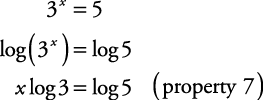Dividing both sides by log 3,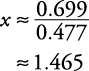Using a calculator for approximation,1.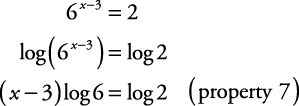Dividing both sides by log 6,Using a calculator for approximation,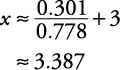1.Using the distributive property,

3 x log 2 – log 2 = 2 x log 3 – 2 log 3

Gathering all terms involving the variable on one side of the equation,

3 x log 2 – 2 x log 3 = log 2 – 2 log 3

Factoring out an x,

x(3 log 2 – 2 log 3) = log 2 – 2 log 3

Dividing both sides by 3 log 2 – 2 log 3,Using a calculator for approximation,

x ≈ 12.770

To solve an equation involving logarithms, use the properties of logarithms to write the equation in the form log bM = N and then change this to exponential form, M = b N .

##### Example 2

Solve the following equations.

1. log 4 (3 x – 2) = 2

2. log 3 x + log 3 ( x – 6) = 3

3. log 2 (5 + 2 x ) – log 2 (4 – x) = 3

4. log 5 (7 x – 9) = log 5 ( x 2x – 29)

1. log 4 (3 x – 2) = 2

Change to exponential form.Check the answer.This is a true statement. Therefore, the solution is x = 6.

1.Change to exponential form.Check the answers.Since the logarithm of a negative number is not defined, the only solution is x = 9.

1. log 2 (5 + 2 x ) – log 2 (4 – x) = 3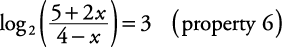Change to exponential form.Using the cross products property,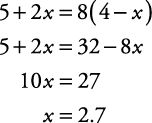Check the answer.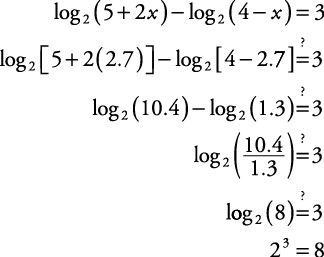This is a true statement. Therefore, the solution is x = 2.7.

1.Check the answers.

If x = 10,This is a true statement.

If x = –2,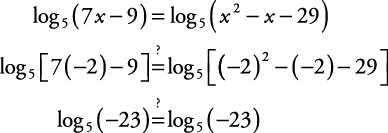This appears to be true, but log 5(–23) is not defined. Therefore, the only solution is x = 10.

##### Example 3

Find log 38.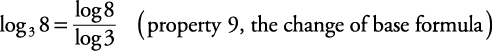Note: log 8 = log 108 and log 3 = log 103.

Using a calculator for approximation,Back to Top
A18ACD436D5A3997E3DA2573E3FD792A